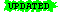Philosophy of Computer Science

# The Halting Problem

## William J. Rapaport

#### Department of Computer Science and Engineering, Department of Philosophy, Department of Linguistics, and Center for Cognitive Science State University of New York at Buffalo, Buffalo, NY14260-2000

 Last Update: 2 February 2010 Note:ormaterial is highlighted

The Halting Problem provides an example of a non-computable function.

Recall that a function is computable if and only if there is an algorithm (i.e., a computer program) that computes it. See "What Is Computation?"

Question:

Is there a computer program (e.g., a program for a Turing machine) that takes as input:

1. a computer program C, and
2. C's input (suppose it's an integer; call it "i")
and that outputs:
• "yes", if C halts on i
• "no", otherwise (i.e., if C loops on i)

Call this program "H".

Since H's input is C and i, we can refer to H and its input as:

H(C, i)

Notes:

1. You can imagine that H(C, i) works as follows:

H gives C its input, i, and then runs C on i.

If C halts on i, then H outputs "yes";
otherwise, H outputs "no".

2. However, we can't answer the question whether C halts on i by just running C on i:

• if it halts, we know it halts, but…

• if it loops, how would we know it loops? (It might just be taking a very long time to halt.)

No! There is no such program H(C, i).

• I.e., H(C, i) is a non-computable function.

Proof (sketch):

Assume by way of contradiction that there is such a program H.

A proof by way of contradiction, or "reductio ad absurdum" (reduction to absurdity), works as follows:

To prove P, assume not-P and derive a contradiction.
Since not-P implies a contradiction, P must have been true.

Now consider another program, H*, which works as follows:

H* is just like H, except that:

• if C halts on i, then H* loops (remember: if C halts on i, then H outputs "yes"), and
• if C loops on i, then H* outputs "no" (just like H does).

Note: If H exists, so does H* (i.e., we can turn H into H* as follows: if H were to output "yes", then let H* go into an infinite loop).

Next, code C as a number, so that it can be treated as input to itself.

Digression:

• This is an insight due to Kurt Gödel.
To code any text, including a computer program, as a number in such a way that you could also decode it, begin by coding each symbol in the text as a unique number (e.g., using ASCII).
Suppose that these numbers, in order, are L1, L2, L3, etc. (where L1 codes the first symbol in the text, L2 codes the second, etc.).
Then compute the following number:

2L1 * 3L2 * 5L3 * 7L4 * …

where each factor is the nth prime raised to the Ln power.
By a theorem of number theory, this can be uniquely factored, the exponents can be recovered, and then they can be decoded to yield the original text.
(Gödel numbering is actually a bit more complicated than this. For more info online, see Godel Numbering or What is Godel's Theorem?.)

• Turing himself has an even simpler way to code symbols; see Turing 1936, §5 ("Enumeration of Computable Sequences").
Now consider H*(C,C). (This step is called "diagonalization".)

I.e., suppose you code up program C as a Gödel number, use it as input to the program C itself, and then let H* do its job on that pair of inputs.

By the definition of H*:

if program C halts on input C, then H*(C,C) loops, and
if program C loops on input C, then H*(C,C) outputs "no".

Now code H* as a number! And consider H*(H*,H*):

In other words, code up H* as a Gödel number, use it as input to the program H* itself, and then let H* do its job on that pair of inputs.

Again, by the definition of H*:

if program H* halts on input H*, then H*(H*,H*) loops, and
if program H* loops on input H*, then H*(H*,H*) outputs "no".

But outputting "no" means that it halts!

So, If H* halts (outputting "no"), then it loops; and if H* loops, then it halts.
It loops if and only if it halts.